# A Guide to SQL Window Functions for Data Analysts

I recently learned about a (somewhat) new feature in MySQL called Window functions. From the perspective of an analyst, Window functions are fantastic: they allow you derive more information about your data without leaving the database. This reduces your need for custom statistical software such as R.

Unfortunately, most of the tutorials that I read on Window functions confused me. I was assigned a very specific problem and was told “Use a Window to function to solve it. Google it.” Google came up with tutorials that were tailored to unrelated problems, and I had to use brute force to shoehorn the tutorials to my specific situation. I do not recommend this approach.

Eventually, things “clicked” and I now feel fluent with Window functions. This guide attempts to get you to my level of fluency in less time by taking a different approach:

1. Start with a sample toy database and ask you to build it on a local machine.
2. Review basic statistical functions in MySQL such as SUM and SUM … GROUP BY.
3. Introduce the “main” Window function OVER(). Combine it with SUM to implement a running total.
4. Introduce other Window functions such as LAG().

In short, this guide presents Window functions as an extension of MySQL’s preexisting statistical functionality. It is also structured as a series of exercises, which you are expected to complete on your own computer. Solutions to all exercises are provided, but you will need to click the “Solution” button to see them. Click the “Solution” button below to see how this works.

Solution

Solutions to exercises will appear here.

If you work with Databases in an analytical capability, then this guide may teach you a new way to extract meaning from your data.

I highly encourage you to run the example code in your own database and attempt the exercises before viewing the solutions. It will help you learn the material much more than simply reading the material.

# Create the Sample Database

All exercises in this Guide are based on this table test.orders. Take a moment and try to describe this dataset.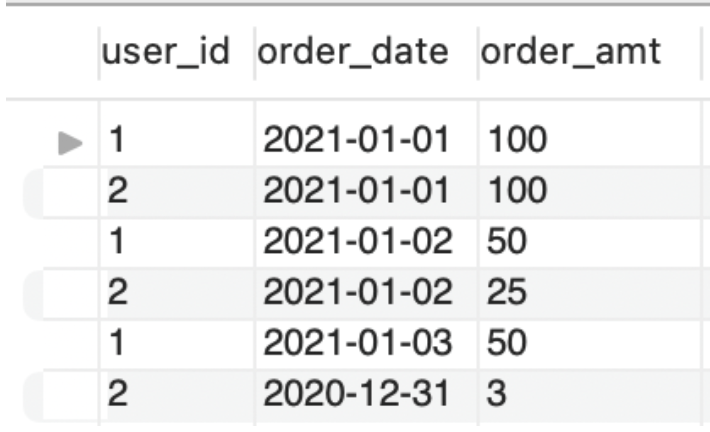Solution

This table shows that two users made a handful of orders. It shows the id of the user who made the purchase, as well as the date and amount purchased.

Create the table on your own machine with this code:

```CREATE DATABASE test;

CREATE TABLE test.orders (
user_id int,
order_date date,
order_amt float
);

INSERT INTO test.orders VALUES
(1, '2021-01-01', 100),
(2, '2021-01-01', 100),
(1, '2021-01-02', 50),
(2, '2021-01-02', 25),
(1, '2021-01-03', 50),
(2, '2020-12-31', 3);

SELECT
*
FROM
test.orders;```

# Basic Analysis (No Window Functions)

Let’s review techniques that you already know for analyzing this table.

### Exercise: ORDER BY

The most basic way to analyze data in SQL is by ordering it. Use ORDER BY to create this table, which orders the data by user_id and order_date. This lets us see, at a glance, who ordered what when: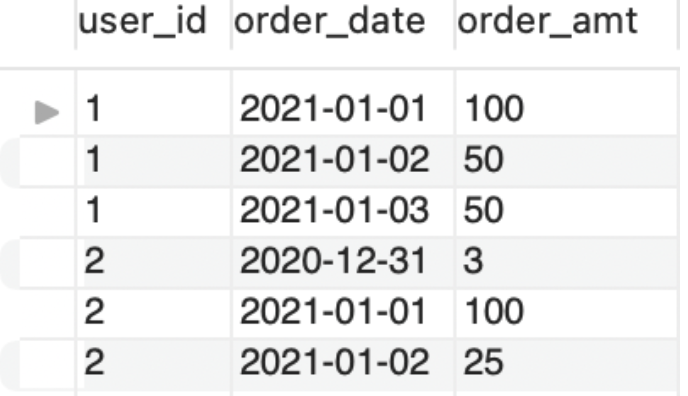Solution
```SELECT
*
FROM
test.orders
ORDER BY
user_id, order_date;```

### Exercise: SUM

The most obvious question to ask is: What is the total amount ordered? Use SUM to create this table:Solution
```SELECT
SUM(order_amt) AS total_ordered
FROM
test.orders;```

### Exercise: SUM … GROUP BY

Until Window functions, GROUP BY was the most powerful statistical tool available in SQL. It allows us to apply functions like SUM to different segments of a table individually.

Use SUM and GROUP BY to create this table, which shows the total amount ordered each day: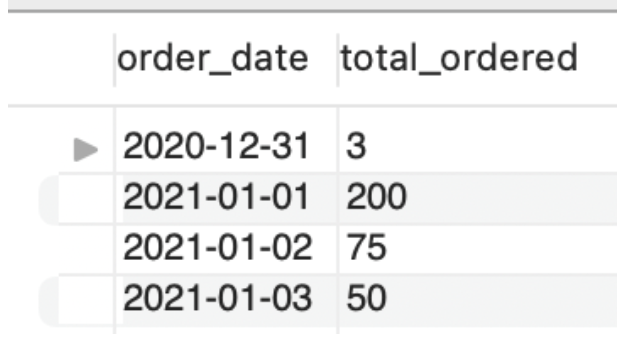Solution
```SELECT
order_date,
SUM(order_amt) AS total_ordered
FROM
test.orders
GROUP BY
order_date
ORDER BY
order_date;```

### Exercise: SUM … GROUP BY

SUM … GROUP BY is very flexible. As an example, create this table, which shows the total amount ordered by each user: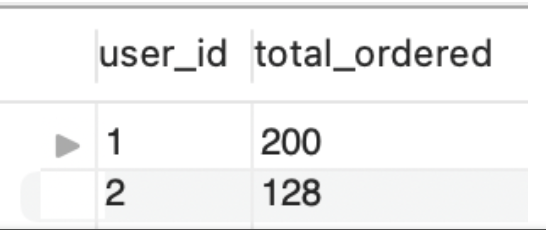Solution
```SELECT
user_id,
SUM(order_amt) AS total_ordered
FROM
test.orders
GROUP BY
user_id
ORDER BY
user_id;```

# Window Functions

### Exercise: Limitations

SUM and SUM … GROUP BY are powerful tools. But they can’t solve every problem you might encounter.

Consider this table. It is the same table that we’ve been using so far, ordered by order_date. But it has a new column at the end: RunningTotal.RunningTotal starts at 3 (the amount of the first order). It ends at 328 (the sum of all orders). For each day in between, it contains the sum of the first day until that day. You might say that the SUM function is being applied over an ever-increasing “Window” of the table.

This column cannot be created with SUM or SUM … GROUP BY. But you should be able to write pseudocode to solve it. This exercise is important, as this pseudocode will serve as a template for the type of problems that Window functions can help you with.

Exercise: write pseudocode for creating the RunningTotal column.

My solution
```For each row i:
RunningTotali = SUM(order_amount0, ..., order_amounti)```

Think of it as applying the SUM function over the order_amount column of all previous rows.

### Exercise: OVER(ORDER BY)

As a reminder, here is my pseudocode for the RunningTotal function:

```For each row i:
RunningTotali = SUM(order_amount0, ..., order_amounti)```

Think of it as applying the SUM function over the order_amount column of all previous rows.

Here is SQL to generating the RunningTotal:

```SELECT
*,
SUM(order_amt) OVER(ORDER BY order_date) AS RunningTotal
FROM
test.orders;```

Enter it into your Console.

As you can see, the “Window function” is called OVER(). Think of it this way “apply the SUM function OVER the table, when the rows are ORDERED BY order_date”.

Because Window functions are applied row by row, we normally specify the order of the rows to apply the function. We do this by sending ORDER BY as a parameter to the OVER function.

### Exercise: Running Average

Using the code from the previous exercise as a template, create this table. In addition to a Running Total, it also contains a Running Average: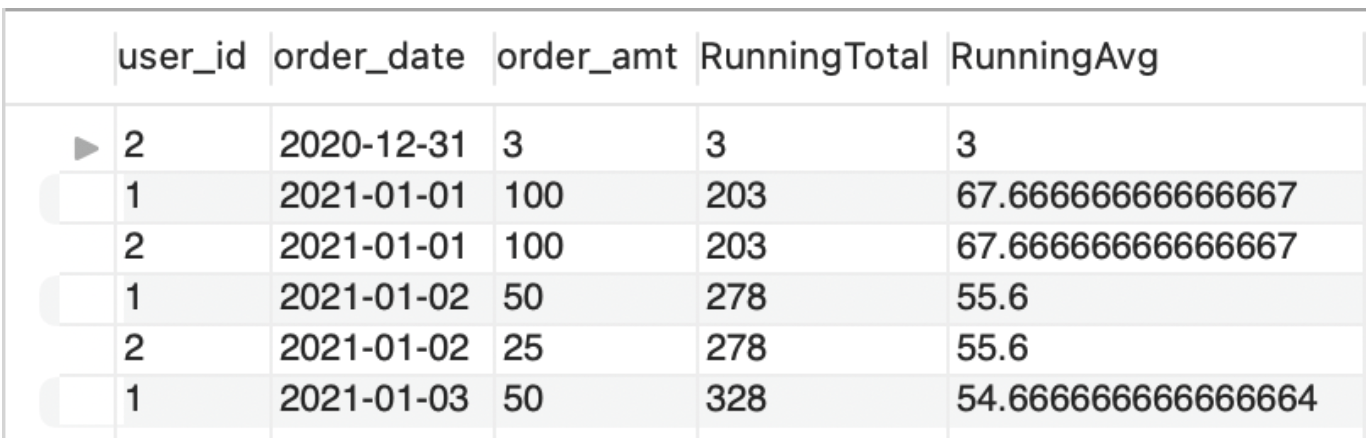Solution:
```SELECT
*,
SUM(order_amt) OVER(ORDER BY order_date) AS RunningTotal,
AVG(order_amt) OVER(ORDER BY order_date) AS RunningAvg
FROM
test.orders;```

If you were able to solve this problem, then you have mastered the basics of Window functions. Let’s review the main points here:

1. The main Window function is called OVER()
2. You use it in a SELECT statement to generate a new column
3. You normally specify the order in which the function is applied to the rows.
4. You can use most statistical (i.e. aggregate) functions with OVER()
5. You can use multiple Window functions in a single statement

The remaining exercises build on this information, and should cover most real-world use cases you will encounter.

### Exercise: ORDER BY … ORDER BY

The syntax of OVER(ORDER BY) was a major stumbling block for me.

For so many years, I had only seen ORDER BY at the end of a SQL statement, where it controls the order in which rows are displayed. An example would be Exercise #1, where rows are ordered first by user_id and then by order_date:

```SELECT
*
FROM
test.orders
ORDER BY
user_id, order_date; ```

But now, with OVER(ORDER BY) it appears that “ORDER BY” might be able to appear twice in a single statement. Is that true? What if they specify different orders? Which would “win”?

The answer is “Yes, ORDER BY can now appear more than once in a single statement. But no, they do not conflict.”

The reason is that the ORDER BY in OVER(ORDER BY) only dictates the order in which a function is applied to rows. It does not control the order in which rows are displayed. In that sense, the ORDER BY at the end of a statement is not impacted by OVER(ORDER BY) at all.

To demonstrate that, create the following table. It displays the RunningTotal in a random order (hint: use RAND()):Solution
```SELECT
*,
SUM(order_amt) OVER(ORDER BY order_date) AS RunningTotal
FROM
test.orders
ORDER BY
RAND();```

### Exercise: OVER(PARTITION BY)

Take a moment to look at this table. How is it similar to previous tables you have already created? How is it different?Solution

It is similar because it is applying the SUM function OVER the table, ORDERED BY order_date.

It is different because it is applying the function once for each user_id.

We previously looked at SUM and SUM … GROUP BY. That is very similar to what is happening here.

The difference is that GROUP BY collapses multiple rows into a single result. There is no “collapsing” here, so we need a different keyword. That keyword is PARTITION BY.

Google something like “SQL PARTITION BY syntax”, and then try to recreate the above table.

Solution
```SELECT
*,
SUM(order_amt) OVER(PARTITION BY user_id ORDER BY order_date) AS RunningTotal
FROM
test.orders;```

# Other Window Functions

So far we’ve talked about show you can use SUM … OVER() to create a running total. We emphasized that OVER() is the main Window function: it applies the “SUM” function to an ever-increasing “Window” of the table.

One reason I chose this example is that I assumed that you already knew about the SUM function. That allowed me to introduce once only once concept at a time (i.e. OVER()).

Another reason is that “create a running total” is a commonly asked question in technical interviews. I.e., it is something you should know how to do if you want to work in this field!

Now that you understand OVER(), we can start looking at functions that can only be used in Window functions.

### Exercise: LAG()

Create this table, which shows, for each order, the date of the previous order by the same user: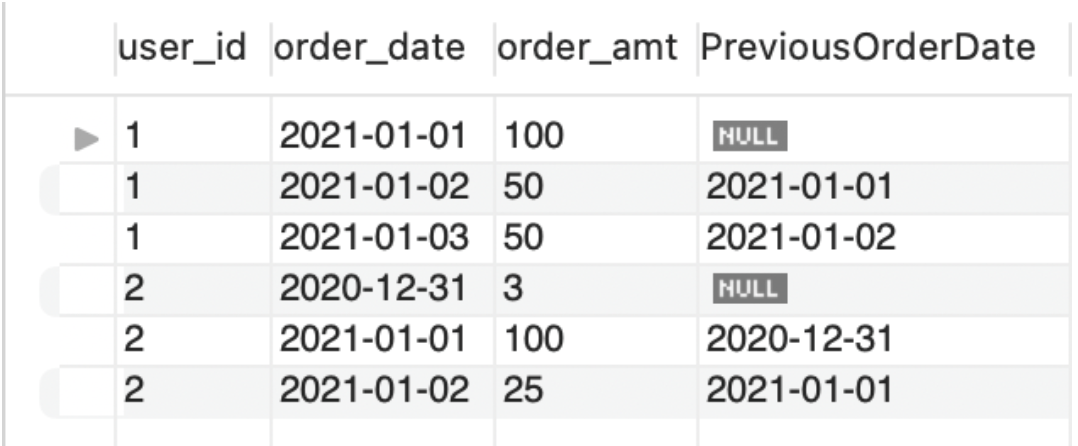You will need to use the LAG() function combined with OVER(). You may want to google “SQL LAG() function”.

Solution
```SELECT
*,
LAG(order_date) OVER(PARTITION BY user_id ORDER BY order_date) PreviousOrderDate
FROM
test.orders
ORDER BY
user_id, order_date;```

Tables like this are frequently created as a precursor to doing an analysis such as the average duration between orders.

### Exercise: All Window Functions

I close by including a screenshot of the official MySQL description of all Window functions (link):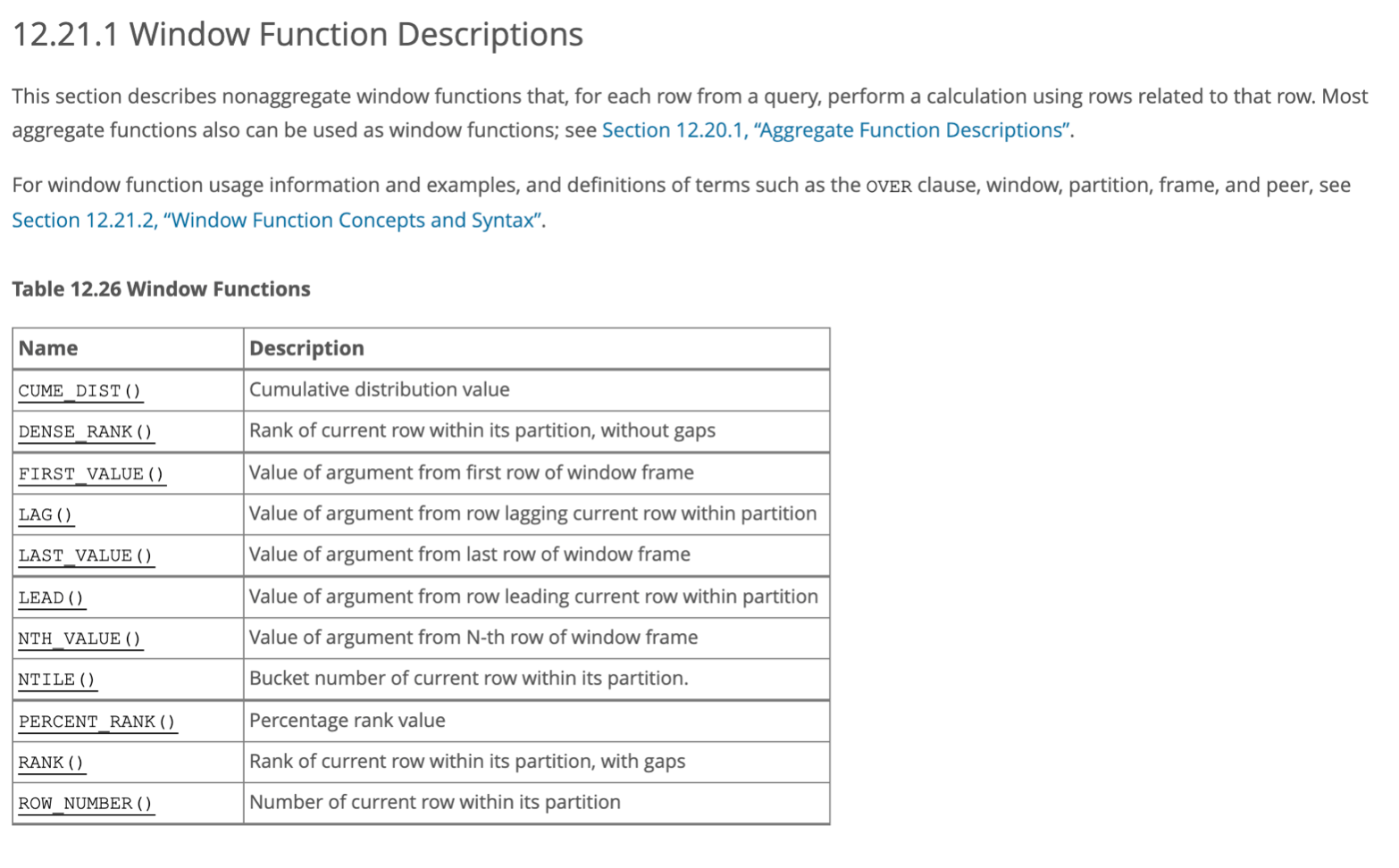Pick one of these functions, and apply it to the our toy database.

# Conclusion

SQL Window functions open up new doors to analysts who work with SQL. As an example, I had previously used the ?cumsum function in R to create a running total of data that resided in MySQL. Now I can do that computation with fairly easy-to-read SQL.

Unfortunately, Window functions took me much longer to master than I’d like to admit. I had specific problems that I needed to solve under a short deadline, most online tutorials were designed for other use cases, and I found the syntax to be confusing.

The way that they finally made sense to me is to view them as an extension to SQL’s existing statistical capabilities. Once SUM … OVER(PARTITION BY … ORDER BY…) made sense to me, all the other Window functions made sense as well. Hopefully this approach / guide helps at least one person become fluent with this feature with less effort than it took me.

If you would like more exercises to learn about Window functions I highly recommend Sylvia Moestl Vasilik’s SQL Practice Problems. I purchased the Professional package, went through all 97 exercises, and it helped me a great deal. I believe that the 57 problems in the Basic package only have one or two exercises with Window functions, but the Professional package contains many more. (I have no financial relationship with Sylvia – I am only recommending her book because I liked it so much).